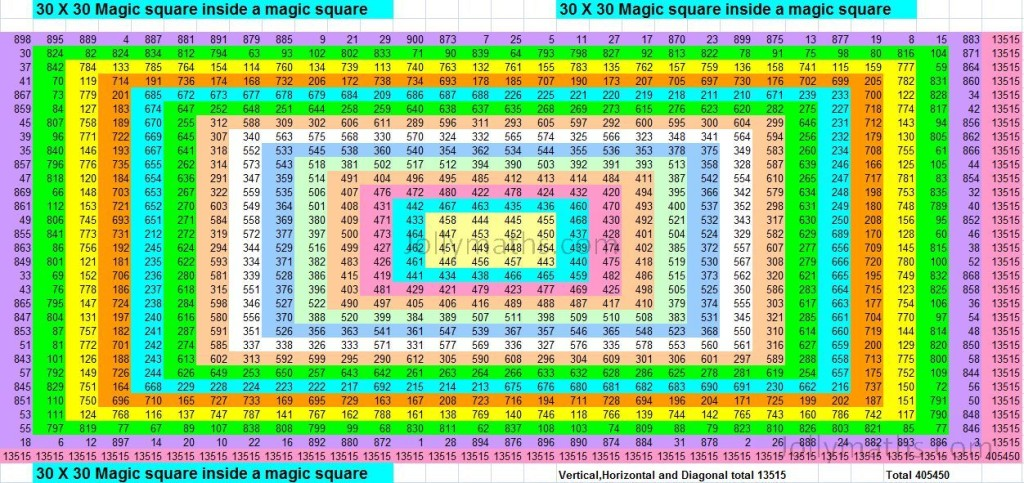# 30 x 30 Magic Squares inside a Magic SquareThe above is a 30 x 30 Magic Squares inside a Magic Square

This is a special construction of a Magic Square wherein we start with a small Magic Square (centermost one in the figure) and added rows and columns around it, ensuring that we have a Magic Square at each stage. This Magic Square has been color-coded to make the Magic Squares clearer.

From the center Yellow coloured is a 4 x 4 Magic square with a total of 1802 in all vertical, Horizontal and in both diagonals. When combininng with the blue coloured sqauare,  that 6 x 6  will give a totl of 2703.  Further it will expand to 8 x 8 , 10 x 10 etc… and finally it will become 30 x 30 with a grand total of 13515.

I have done this Magic squares inside the magic square from 5 x 5,     6 x 6, 7 x 7, …….198 x 198, 199 x 199 and 200 x 200.  In the 200 x 200 Magic squares inside a magic square all the numbers from 1 to 40,000 are used and the row total will be 4,00,00,100.  Also I am  planning to do it upto 1500 x 1500.

Definitely, it is a gift given to me by Almighty.  I thank the Almighty for giving me the idea, guided me to work, and helped me to complete it with much difficulties.

# SWASTIKA Magic Square

This is a special Magic Square called as the SWASTIKA Magic Square. All odd numbers are inside the SWASTIKA SYMBOL. (The Magic-Total is 65)

[table id=swastika-5×5 /]

You can find more special Magic-Squares here

# Magic Squares – What are they?

Magic squares are a set of numbers filled in a 3 x 3, 4 x 4,… squares and after filling it, all the vertical, Horizontal and both diagonal totals are equal.

Properties of the Magic Square:

1. Magic squares are made in 3 x 3, 4 x 4, 5 x 5….etc.
2. Generally a 3 x 3 magic square is filled with numbers 1 to 9 and 4 x 4 magic square is filled with 1 to 16,…… In general a “n x n” magic square will be filled with 1 to n square numbers.
3. In general in a “n x n” magic square will have “n” rows and “n” columns and n square Small squares.
4. After filling all squares  ALL VERTICAL, ALL HORIZONTAL AND BOTH DIAGONAL THE TOTALS ARE EQUAL.
5. A magic square done from 1 to 9, 1 to 16,… are called as Base Magic square.
6. The total of a row/column of a Base Magic Square will be “First number plus the Last number added together, divided by two, and then multiplied by the Number of rows”. i.e. {( 1 + n square ) / 2} ……. {( 1 + n square ) divided 2}
7. Generally natural numbers 1,2,3,… are used for forming the magic squares and we can use fractions, zero, negative numbers and squares, cubes etc etc…
8. We can make 3 x 3, 4 x 4, 5 x 5… upto infinite.  Magic squares for a particular number, particular size and for a given space interval is at our choice.
9. Also we can make magic squares for a given number, given year or given date of birth etc.